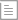资讯

# proe模具画法，教你如何画好齿轮零件

2018-08-30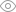6855 分类：结构工程

proe模具画法，教你如何画好齿轮零件

/* 为笛卡儿坐标系输入参数方程

/*根据t (将从0变到1) 对x, y和z

/* 例如:对在 x-y平面的一个圆，中心在原点

/* 半径 = 4，参数方程将是:

/*           x = 4 * cos ( t * 360 )

/*           y = 4 * sin ( t * 360 )

/*           z = 0

/*-------------------------------------------------------------------

alpha=20

m=0。8

z=24

r0=0。5*m*z*cos(20)

t0=t*40

x0=(cos(t0)+t0*pi/180*(sin(t0)))*r0

y0=(sin(t0)-t0*pi/180*(cos(t0)))*r0

theta=-(tan(alpha)-alpha*pi/180)*180/pi-90/z

x=x0*cos(theta)-y0*sin(theta)

y=x0*sin(theta)+y0*cos(theta)

z=0

齿轮模数M=0。8，齿轮齿数Z=24

齿顶圆∅=齿轮模数M * (齿轮齿数Z+2)=20。8

齿根圆∅=齿轮模数M * (齿轮齿数Z-2或2。1)=17。52

单齿角度2A=360/(24公齿+24母齿)=7。5°

2齿夹角B=单齿角度2A * 2=15°1。根据现有参数画出齿轮最大外围(齿顶圆)的拉伸体，注意该拉伸体须以Front为草绘平面(坐标系的XY平面，Z向上)，并作出齿轮方程线

2。画出齿轮的分度圆，齿根圆。找出分度圆与方程线的交点，连此交点到圆心做一直线L1,再以单齿半角A3。75°作出该齿的中心线L2,以L2为镜像中心线镜像齿轮方程线

3。延长两方程线至齿根圆，通过修剪完成单齿轮廓线。

4。通过单齿轮廓线拉伸切除阵列完成齿轮外形。1。根据现有参数画出齿轮最大外围(齿顶圆)的拉伸体，注意该拉伸体须以Front为草绘平面(坐标系的XY平面，Z向上)，并作出齿轮方程线

2。画出齿轮的齿根圆，直接以穿过圆心的水平线做镜像中心线镜像齿轮方程线，再以两邻齿夹角B15°为旋转复制角度对两齿轮方程线进行旋转复制。

3。 延长4根方程线中间的2根至齿根圆，通过修剪完成单齿轮廓线。

4。通过单齿轮廓线拉伸切除阵列完成齿轮外形。

1。D(分度圆直径)=M(齿轮模数) * Z(齿轮齿数)

2。D1(齿顶圆直径)= M * (Z+2)

3。D2(齿根圆直径)= M * (Z-2～2。1)

1。当取值2时，两吻合齿轮无间隙

2。当取值2。05时，两吻合齿轮有一定间隙

3。当取值2。1时，两吻合齿轮有较大间隙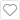收藏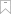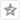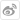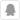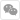Rhino

keyshot

Pro/Engineer

Photoshop

IF概念学生设计大赛

# 官方微信400-088-6822